# LC Circuit Analysis: Series And Parallel Circuits, Equations And Transfer Function

Contents

## What is an LC Circuit?

An LC circuit (also known as an LC filter or LC network) is defined as an electrical circuit consisting of the passive circuit elements an inductor (L) and a capacitor (C) connected together. It is also called a resonant circuit, tank circuit, or tuned circuit.

Due to the absence of a resistor in the ideal form of the circuit, an LC circuit consumes no energy. This is unlike the ideal forms of RC circuits, RL circuits, or RLC circuits, which consume energy due to the presence of a resistor.

That said in a practical circuit, an LC circuit will always consume some energy because of the non-zero resistance of the components and connecting wires.

### Why an LC Circuit is Called a Tuned Circuit or Tank Circuit?

The charge flows back and forth between the plates of the capacitor and through the inductor. The energy oscillates between a capacitor and an inductor until the internal resistance of the components and connecting wires makes the oscillations die out.

The action of this circuit is like a tuned action, mathematically known as a harmonic oscillator, which is similar to a pendulum swinging back and forth or water flowing back and forth in a tank; for this reason, the circuit is called tuned circuit or tank circuit.

The circuit can act as an electrical resonator and storing energy oscillating at the frequency called a resonant frequency.

## Series LC Circuit

In the series LC circuit, the inductor and capacitor both are connected in a series that is shown in the figure.

Since in a series circuit current is the same everywhere in the circuit hence the flow of current is equal to the current through both the inductor and the capacitor.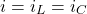Now the total voltage across the terminals is equal to the sum of the voltage across the capacitor and the voltage across the inductor.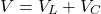### Resonance in Series LC Circuit

When a frequency increases the magnitude of the inductive reactance also increases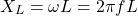and the magnitude of the capacitive reactance decreases.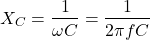Now at a resonance condition a magnitude of both inductive reactance and capacitive reactance becomes equal.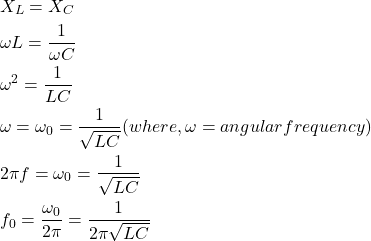Where,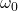is a resonant angular frequency (radians per second).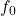is a resonant frequency (Hertz).

Now an impedance of the series LC circuit is given by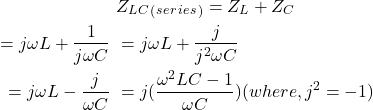Now the angular resonant frequency is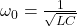, then impedance becomes

(1)Thus at resonant condition when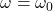total electrical impedance Z will be zero means XL and XC cancel out each other. hence, current supplied to a series LC circuit is maximum (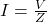).

Therefore the series LC circuit, when connected in series with the load, will act as a band-pass filter having zero impedance at the resonant frequency.

• At frequency below resonant frequency i.e.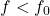,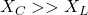. Hence the circuit is capacitive.
• At frequency above resonant frequency i.e.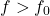,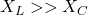. Hence the circuit is inductive.
• At resonant frequency i.e.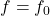,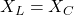. the current is maximum and impedance is minimum. In this state, the circuit can act as an acceptor circuit.

## Parallel LC Circuit

In the parallel LC circuit, the inductor and capacitor both are connected in parallel that is shown in the figure.

The Voltage across each terminal of different elements in a parallel circuit is the same. Hence the voltage across the terminals is equal to the voltage across the inductor and the voltage across the capacitor.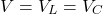Now the total current flowing through the parallel LC circuit is equal to the sum of the current flowing through the inductor and the current flowing through the capacitor.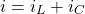### Resonance in Parallel LC Circuit

At resonance condition when the inductive reactance (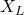) equal to the capacitive reactance (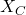), the reactive branch current is equal and opposite. Hence, they cancel out each other to give minimum current in the circuit. In this state total impedance is maximum.

The resonant frequency is given by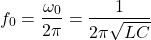Now an Impedance of the Parallel LC circuit is given by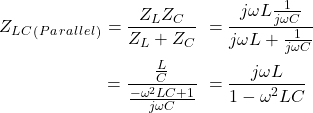Now the angular resonant frequency is, then impedance becomes

(2)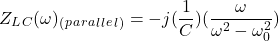Thus at resonant condition whentotal electrical impedance Z will be infinite and current supplied to a parallel LC circuit is minimum ().

Therefore the parallel LC circuit, when connected in series with the load will act as a band-stop filter having infinite impedance at the resonant frequency. The parallel LC circuit connected in parallel with the load will act as a band-pass filter.

• At frequency below resonant frequency i.e. f<f0, XL >> XC. Hence the circuit is inductive.
• At frequency above resonant frequency i.e. f>f0, XC >> XL. Hence the circuit is capacitive.
• At resonant frequency i.e. f = f0, XL = XC, the current is minimum and impedance is maximum. In this state, the circuit can act as a rejector circuit.

## LC Circuit Equations

### Current and voltage equation

• At initial condition: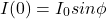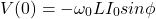• At oscillation: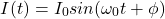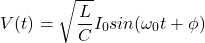### LC circuit differential equation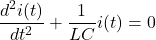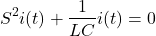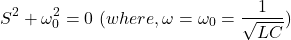### Impedance of the Series LC circuit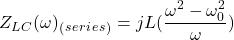### Impedance of the Parallel LC circuit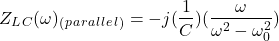#### Setting Time

The LC circuit can act as an electrical resonator and storing energy oscillates between the electric field and magnetic field at the frequency called a resonant frequency. Since any oscillatory system reaches in a steady-state condition at some time, known as a setting time.

The time required for the response to decreasing and becomes steady at its steady-state value and remains thereafter within +- 2% of its final value is called setting time.

### LC Circuit Current

Assume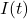is the instantaneous current flowing through the circuit. The voltage drop across the inductor is expressed in terms of current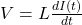and the voltage drop across the capacitor is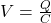, where Q is the charge stored on the positive plate of the capacitor.

Now according to Kirchhoff’s voltage law, the sum of potential drops across the various components of a closed-loop is equal to zero.

(3)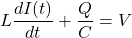Dividing the above equation by L and differentiating it with respect to t, we get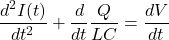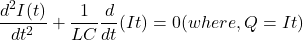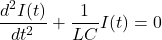(4)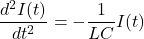Now the current in a simple harmonic oscillations form is given by:

(5)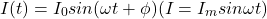Where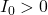andare constants.

Put the value of equation (5) into (4) we get,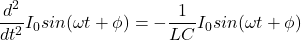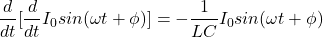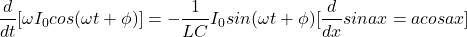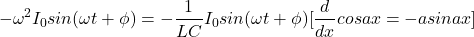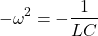(6)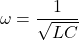Thus from the above equation, we can say that the LC circuit is an oscillating circuit and it oscillates at a frequency called a resonant frequency.

### LC Circuit Voltage

Now according to equation (3), the induced voltage across an inductor is minus the voltage across the capacitor.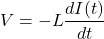Put the equation of current from equation (5), we get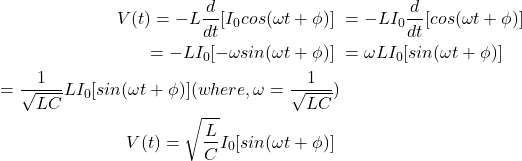In other words, the voltage reaches the maximum when the current reaches zero and vice versa. The amplitude of voltage oscillation is that of the current oscillation multiplied by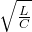.

## Transfer Function of LC Circuit

The transfer function from the input voltage to the voltage across capacitor is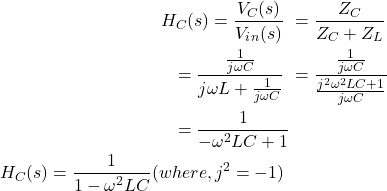Similarly, the transfer function from the input voltage to the voltage across inductor is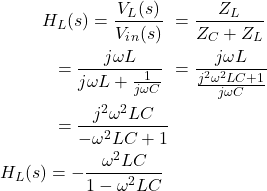## Natural Response of LC Circuit

Let us assume that the capacitor is initially fully discharged and switch (K) is kept open for a very long time and it is closed at t=0.

• At t=0switch K is open

This is an initial condition hence we can write,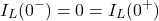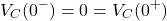Because the current through the inductor and the voltage across the capacitor cannot change instantaneously.

• For all t>=0+ switch K is closed

Now the voltage source is introduced in the circuit. Hence applying KVL to the circuit, we get,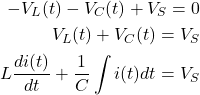Here voltage across the capacitor is expressed in terms of current.

The above equation is called the integro-differential equation. Differentiating both sides of the above equation with respect to t, we get,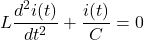(7)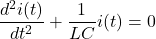Equation (7) indicates a second-order differential equation of an LC circuit.

Replace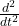with s2, we get,

(8)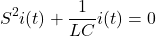Now roots of the above equation is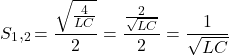Here,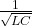is the natural frequency of oscillation.

### LC Circuit Frequency Response

Using Impedance method: General equation for frequency response system is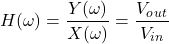• Assume that the output voltage occurs across the capacitor terminals, apply potential divider rule to the above circuit

(9)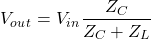Where,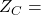Impedance of the capacitor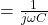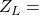Impedance of the inductor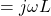Substitute it in equation (9), we get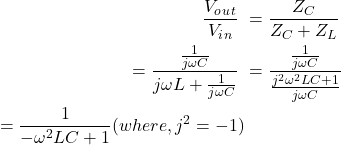(10)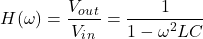• Assume that the output voltage occurs across the inductor, apply potential divider rule to the above circuit

(11)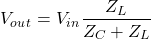Substitute value of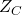and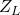in above equation, we get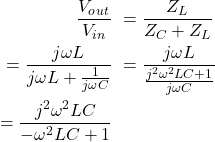(12)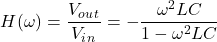The equation (10) and (12) indicates the frequency response of an L-C circuit in complex form.

## LC Circuit Differential Equation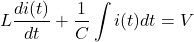The above equation is called the integro-differential equation. Here voltage across the capacitor is expressed in terms of current.

Now, differentiating above equation both sides with respect to t, we get,(13)The above equation indicates the second-order differential equation of LC circuit.

Replacewith s2, we get,

(14)Now,therefore,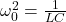, put it in above equation we get,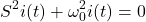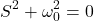## LC Circuit Charging and Discharging

In an LC circuit the inductor and the capacitor both are storing elements i.e. inductor stores energy in its magnetic field (B), depending on the current through it, and capacitor stores energy in the electric field (E) between its conducting plates, depending on the voltage across it.

Assume that initially, the capacitor contains a charge q, and then all the energy of the circuit is initially stored in the electric field of the capacitor. The energy stored in the capacitor is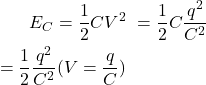Now if an inductor is connected across a charged capacitor, the voltage across the capacitor will cause current to flow through the inductor, which produces a magnetic field around the inductor, the capacitor starts discharging and the voltage across the capacitor reduces to zero as the charge is used up by the current flow (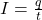).

Now the capacitor is completely discharged and all the energy is stored in the magnetic field of the inductor. At this instant, the current is at its maximum value and the energy stored in the inductor is given by (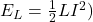.

Due to the absence of a resistor, there is no energy dissipates in the circuit. Thus, the maximum energy stored in the capacitor is equal to the maximum energy stored in the inductor.

At this instant stored energy in the magnetic field around an inductor induces a voltage across the coil according to the faraday’s law of electromagnetic induction (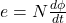). This induced voltage causes a current to flow through the capacitor and the capacitor begins to recharge with a voltage of opposite polarity.

This charging and discharging process will begin again, with the current flowing in the opposite direction through the inductor as before.

Thus charging and discharging of the LC circuit can be in a cyclic manner and energy oscillates back and forth between the capacitor and the inductor until the internal resistance makes the oscillations die out.

The figure shows the charging and discharging voltage and current waveform.

## LC Circuit Applications

The applications of LC Circuits include:

• The applications of an LC circuit mainly involve in many electronic devices, particularly radio equipment such as transmitters, radio receivers, and TV receivers, amplifiers oscillators, filters, tuners, and frequency mixers.
• LC circuits are also used for producing signals at a particular frequency or accepting a signal from a more complex signal at a particular frequency.
• The main purpose of an LC circuit is usually to oscillate with minimum damping, so the resistance is made as low as possible.
• A series resonance circuit provides voltage magnification.
• A parallel resonance circuit provides current magnification.

### What is Damping?

Damping is the decrease in the amplitude of an oscillation or wave motion with time. Resonance is the increase of amplitude as damping decreases.

Want To Learn Faster? 🎓
Get electrical articles delivered to your inbox every week.
No credit card required—it’s 100% free.